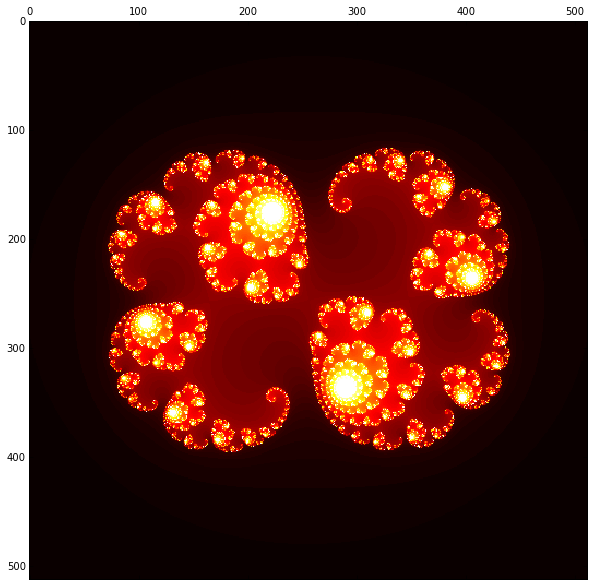# DistArray Julia Set¶

The Julia set, for a given complex number $c$, is the set of points $z$ such that $|z_{i}|$ remains bounded where $z_{i+1} = z_{i}^2 + c$.

This can be plotted by counting how many iterations are required for $|z_{i}|$ to exceed a cutoff.

Depending on the value of $c$, the Julia set may be connected and contain a lot of points, or it could be disconnected and contain fewer points. The points in the set will require the maximum iteration count, so the connected sets will usually take longer to compute.

First, some imports.

In :
from __future__ import print_function

import numpy
import matplotlib.pyplot as plt

# DistArray imports
from distarray.globalapi import Context, Distribution
from distarray.globalapi.distarray import DistArray

# inline plots
%matplotlib inline

# bigger figures
plt.rcParams.update({'figure.figsize': (10, 10)})


## Julia set kernels¶

To avoid round-trips between the client and engines, our strategy will be to push a function to each engine to compute that engine's local section of the Julia set. Here we have two options for this "kernel": a version that avoids NumPy fancy indexing, and one that uses it.

In :
def numpy_julia_calc(z, c, z_max, n_max):
"""Calculate the Julia set using NumPy.

Parameters
----------
z : NumPy array
array of complex values whose iterations we will count.
c : complex
Complex number to add at each iteration.
z_max : float
Magnitude of complex value that we assume goes to infinity.
n_max : int
Maximum number of iterations.
"""
z = numpy.asarray(z)
counts = numpy.zeros_like(z, dtype=numpy.int32)
hits = numpy.zeros_like(z, dtype=numpy.bool)
n = 0

while not numpy.all(hits) and n < n_max:
z = z * z + c
mask = (abs(z) > z_max) & (~hits)
z[hits] = 0
n += 1
counts[~hits] = n_max
return counts

def fancy_numpy_julia_calc(z, c, z_max, n_max):
"""Calculate the Julia set using NumPy fancy indexing.

Parameters
----------
z : NumPy array
array of complex values whose iterations we will count.
c : complex
Complex number to add at each iteration.
z_max : float
Magnitude of complex value that we assume goes to infinity.
n_max : int
Maximum number of iterations.
"""
z = numpy.asarray(z)
counts = numpy.zeros_like(z, dtype=numpy.int32)
hits = numpy.zeros_like(z, dtype=numpy.bool)
n = 0

while not numpy.all(hits) and n < n_max:
z[~hits] = z[~hits] * z[~hits] + c
mask = (abs(z) > z_max) & (~hits)
n += 1
counts[~hits] = n_max
return counts


## Coordinating functions¶

Here we have functions that create a DistArray representing the complex plane and functions that coordinate applying the kernel on each distributed section of the DistArray.

In :
def create_complex_plane(context, resolution, dist, re_ax, im_ax):
"""Create a DistArray containing points on the complex plane.

Parameters
----------
context : DistArray Context
resolution : 2-tuple
The number of points along Re and Im axes.
dist : 2-element sequence or dict
dist_type for of the DistArray Distribution.
re_ax : 2-tuple
The (lower, upper) range of the Real axis.
im_ax : 2-tuple
The (lower, upper) range of the Imaginary axis.
"""

import numpy as np

def fill_complex_plane(arr, re_ax, im_ax, resolution):
"""Fill in points on the complex coordinate plane."""
re_step = float(re_ax - re_ax) / resolution
im_step = float(im_ax - im_ax) / resolution
for i in arr.distribution.global_iter:
for j in arr.distribution.global_iter:
arr.global_index[i, j] = complex(re_ax + re_step * i,
im_ax + im_step * j)

# Create an empty distributed array.
distribution = Distribution(context, (resolution, resolution),
dist=dist)
complex_plane = context.empty(distribution, dtype=np.complex64)
context.apply(fill_complex_plane,
(complex_plane.key, re_ax, im_ax, resolution))
return complex_plane

In :
def local_julia_calc(la, c, z_max, n_max, kernel):
"""Calculate the number of iterations for the point to escape.

Parameters
----------
la : LocalArray
LocalArray of complex values whose iterations we will count.
c : complex
Complex number to add at each iteration.
z_max : float
Magnitude of complex value that we assume goes to infinity.
n_max : int
Maximum number of iterations.
kernel : function
Kernel to use for computation of the Julia set.  Options are 'fancy',
'numpy', or 'cython'.
"""
from distarray.localapi import LocalArray
counts = kernel(la, c, z_max, n_max)
res = LocalArray(la.distribution, buf=counts)
return proxyize(res)  # noqa

def distributed_julia_calc(distarray, c, z_max, n_max,
kernel=fancy_numpy_julia_calc):
"""Calculate the Julia set for an array of points in the complex plane.

Parameters
----------
distarray : DistArray
DistArray of complex values whose iterations we will count.
c : complex
Complex number to add at each iteration.
z_max : float
Magnitude of complex value that we assume goes to infinity.
n_max : int
Maximum number of iterations.
kernel: function
Kernel to use for computation of the Julia set.  Options are 'fancy',
'numpy', or 'cython'.
"""
context = distarray.context
iters_key = context.apply(local_julia_calc,
(distarray.key, c, z_max, n_max),
{'kernel': kernel})
iters_da = DistArray.from_localarrays(iters_key, context=context,
dtype=numpy.int32)
return iters_da


## Using DistArray to explore the Julia set¶

In :
context = Context()  # handles communicating with the engines

In :
# create the complex plane
resolution = (512, 512)
dist = 'cc'  # different distributions of the data among the engines
# one character per dimension
# 'c' stands for 'cyclic', 'b' for block
re_ax = (-1.5, 1.5)
im_ax = (-1.5, 1.5)
complex_plane = create_complex_plane(context, resolution, dist, re_ax, im_ax)

# calculate the Julia set
z_max = 2.0
n_max = 100
c = complex(0.285, 0.01)
iters_da = distributed_julia_calc(complex_plane, c, z_max, n_max, kernel=numpy_julia_calc)

# plot it!
plt.matshow(iters_da.toarray(), cmap='hot')

Out:
<matplotlib.image.AxesImage at 0x1091abf90>In :
context.close()